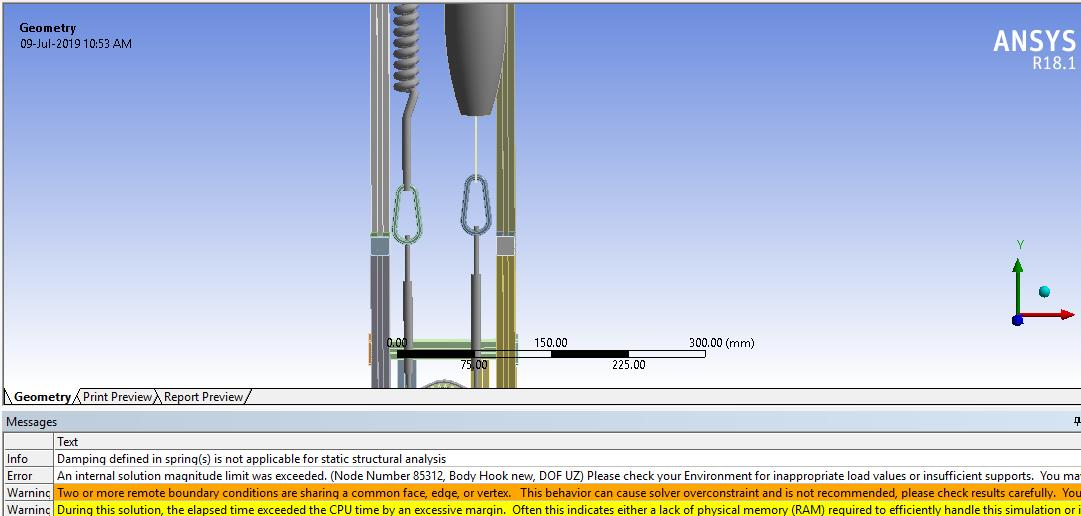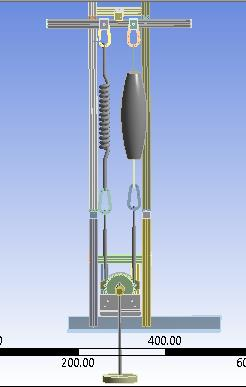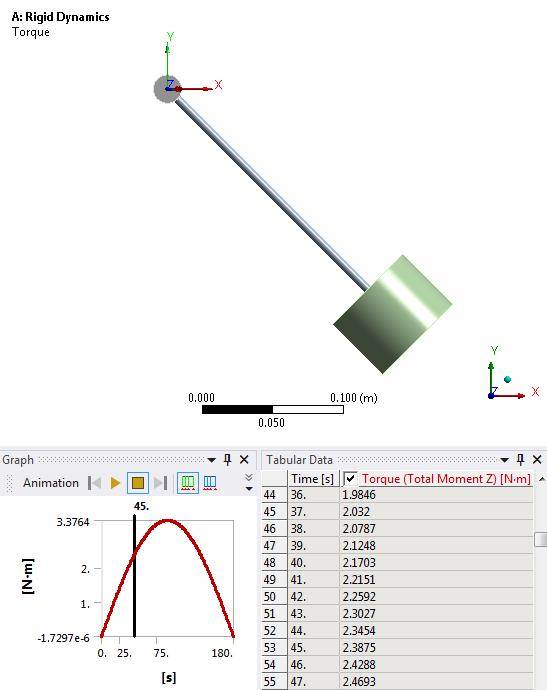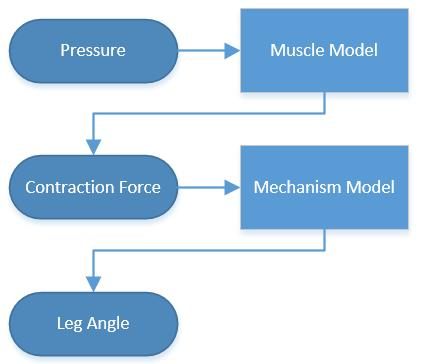## General Mechanical

•shantashreejena97
Subscriber

Hello everyone,

I have hung a hook with two springs at its 2 end. but it shows error while solving saying the hook has a degree of freedom in the Z direction. What could I do to do it fully constraint? In the figure(below) left side hook has an error in contact which is connected to spring in both sides. I have also attached the figure of my whole assembly for your further reference.•peteroznewman
Subscriber

Hello Shantashree,

It is helpful to sketch an ideal abstraction of a mechanism, showing the minimum amount of detail required to produce the desired motion. A stick-figure sketch is often what a simulation is modeled after, because it is so simple, yet represents the essential characteristics of the problem.

A physical prototype has a lot more detail that is not represented in the sketch because there are extra parts needed to hold things together, but those extra parts are not relevant to the basic function of the mechanism or structure. I would put the oval-shaped hooks in this category. Why are the hooks important to the question you want to answer. I would reframe your question and say the answer is not to constrain the hooks but to remove them from the model.

Please reply with a simple sketch that shows with a minimum number of parts, the loads, supports and motion you want to simulate. Describe what result you want from the simulation.

Regards,
Peter

•shantashreejena97
Subscriber

Hi Peter,

Actually, I want to show at a certain compressive force that acts on both the top and bottom face of muscle how many angles would the weight be lifted (taking the weight of the weight into consideration)? If I remove them from my model then with whom the spring and muscle will be attached to?

•peteroznewman
Subscriber

Hi Shantashree,

If you built the simple Rigid Dynamics model I suggested in this post, then you have a graph of Torque vs Lift Angle. You can multiply the Torque by the sprocket radius to get Force.

The idea is that you don't need to connect a spring element to a hook, that is hanging from the frame, you remove the hook and connect the spring element to the frame directly. The hook isn't essential to the model. Similarly, you don't need a muscle if you apply the force the muscle generates to the part that it is pulling on.

•shantashreejena97
Subscriber

Then in place of the muscle what should I put to show that there is equal and opposite force on both side that compress the muscle and thereby the chain(here another spring) lifted up which further rotates the sprocket and also creates a tensile force on the spring. The sprocket is also fixed to the shaft. With the rotation of the shaft, the rod and weight lifted to some angle which I want to know. And also should I remove bottom two hooks which are attached to both the chain(here rigid spring)? If yes then with whom I connect the chain to?

•peteroznewman
Subscriber

Here is a graph of Torque vs Lift Angle created from a Rigid Dynamics Model that has no spring and no muscle. The model has a Gravity load and a revolute to drive the rotation by 180 degrees. If gravity is g, the mass is m and the radius is r then the Torque is m.g.r.sin(theta).At 37 degrees, it takes 2.0 N-m of torque to hold the mass at that angle.  If the sprocket puts the chain at a 40 mm radius, then the tension in the chain and hence the tension in the muscle to hold the weight at 38 degrees is 2.0/0.040 = 50 N for a system with no spring on the other side of the sprocket (neglecting the weight of the chain).

Is it important to apply the 50 N to the frame where the muscle hangs from the top hook?  If so, you don't need the muscle or the hook, you apply 50 N downward to where the hook was hanging.

If you want to add the effect of the spring on the other side of the sprocket to the muscle, then you need to define the spring preload P at zero degrees of rotation and the spring rate k in N/mm so you can calculate the spring tension F as a function of extension x relative to zero degrees, which is F = P + kx.

You can convert that equation into Torque as a function of angle because you know the sprocket radius is 40 mm. That torque is added to the torque to lift the mass, which you can copy from the results table in the simple model. You can sum two Torque columns in Excel and convert that back to a force in the muscle in another column.

•shantashreejena97
Subscriber

Actually, in real life situation, we are giving pressure to the muscle which creates an expansion of it by which respective elements lifted which helps to lift the weight by some angle. This is what I want to know in this analysis. That's why muscle is important in this analysis. Can't we do analysis with muscle?. Because it is important for us to show the muscle expansion. That's why I thought of instead of giving pressure to give compressive force to both ends of the muscle.

•peteroznewman
Subscriber

The whole system is divided into two subsystems because each subsystem model is more easily built than a whole system model and the interface between the subsystems is easily defined.  I defined the Mechanism Model above. You can build a Muscle Model next.